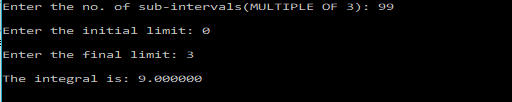# Simpson’s 3/8th Rule – C PROGRAM

Simpson’s 3/8th Rule is a Numerical technique to find the definite integral of a function within a given interval.
It’s so called because the value 3/8 appears in the formula.

The function is divided into many sub-intervals and each interval is approximated by a cubic curve. And the area is then calculated to find the integral. The more is the number of sub-intervals used, the better is the approximation.

### FORMULA:$\int_a^b f(x)dx= \frac{3h}{8}(f_0 + 3f_1 + 3f_2 + 2f_3 + 3f_4 + 3f_5 + 2 f_6 ....+ 3f_{n-1} + f_n)$
where,$f_i=a+ih$ where$i$ starts from 0 and goes to$n$
NOTE: The no. of sub-intervals$n$, should be a multiple of 3 for this method.

### PROGRAM:

/*********************************
*******SIMPSON'S 3/8 RULE********
********************************/
#include<stdio.h>
#include<math.h>
double f(double x){
return x*x;
}
main(){
int n,i;
double a,b,h,x,sum=0,integral;
printf("\nEnter the no. of sub-intervals(MULTIPLE OF 3): ");
scanf("%d",&n);
printf("\nEnter the initial limit: ");
scanf("%lf",&a);
printf("\nEnter the final limit: ");
scanf("%lf",&b);
h=fabs(b-a)/n;
for(i=1;i<n;i++){
x=a+i*h;
if(i%3==0){
sum=sum+2*f(x);
}
else{
sum=sum+3*f(x);
}
}
integral=(3*h/8)*(f(a)+f(b)+sum);
printf("\nThe integral is: %lf\n",integral);
}


### OUTPUT:[wpedon id="7041" align="center"]

## One thought on “Simpson’s 3/8th Rule – C PROGRAM”

1. Thank you
Very good

It my hehp

best regards

Prof. Moises Meza Pariona

State University of Ponta Grossa-PR, Brazil
[email protected]